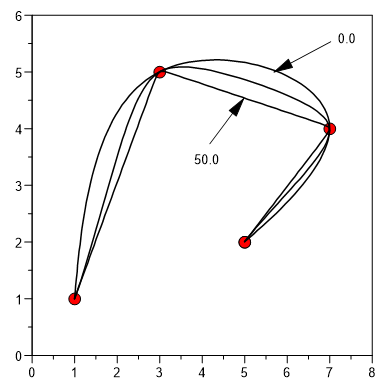# pspline¶

pspline computes a spline under tension for a given x and y vector. In contrast to the fspline function, the x-elements do not have to be sorted in increasing order.

`rmXY = pspline(rvX, rvY)`
`rmXY = pspline(rvX, rvY, rsTensionFactor)`
`rmXY = pspline(rvX, rvY, rsTensionFactor, nPoints)`

Return Value

rmXY is a real matrix with two columns. The first column contains the x-coordinates of the spline and the second column contains the y-coordinates of the spline.

Parameters

`rvX`

rvX is a real vector with at least 3 elements.

`rvY`

rvY is a real vector with at least 3 elemetns.

`rsTensionFactor`

rsTensionFactor is a value in the range 0 to 50. For 0 the spline is similar to a cubic spline and for values close to 50 the result is a polygon.

`nPoints`

nPoints is the number of points of rmXY.

Example```x = [1,3,7,5]
y = [1,5,4,2]
h = plot(x,y)
xy = pspline(x,y,0)
plot(xy[;1],xy[;2],h)
xy = pspline(x,y,5)
plot(xy[;1],xy[;2],h)
xy = pspline(x,y,50)
plot(xy[;1],xy[;2],h)
```